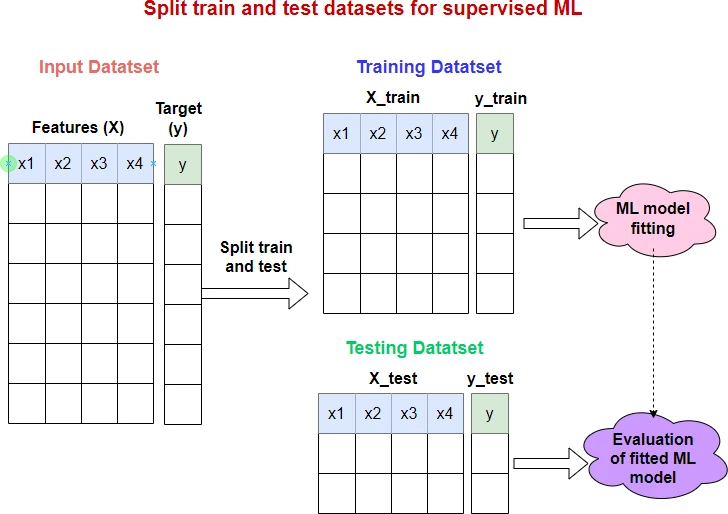# How to split train and test datasets in Python (using sklearn)

In a supervised machine learning (ML) framework, the train and test split of input datasets is a key method for unbiased evaluation of the predictive performance of the fitted (trained) model.

The train and test split method generate two disjoint datasets i.e. training and testing datasets. The training dataset is used for fitting (training) the ML model, and the testing dataset (that is not used in the fitting model) is used for evaluating the performance of the fitted model.A common train and test splitting ratio is 80:20 (80% training size, 20% testing size). The other ratios that are also used in practice are 75:25, 70:30, 60:40, and sometimes even 50:50. A good splitting ratio depends on the input dataset, but there is no clear guidance on how to choose it.

The splitting of the input datasets is helpful in the unbiased evaluation and avoids overfitting of the model.

## How to perform train and test split in Python

In python, the train and test split can be performed using the `train_test_split()` function from the scikit-learn package. This function is available in `sklearn.model_selection` module.

The basic syntax of `train_test_split()` is as follows:

``````X_train, X_test, y_train, y_test = sklearn.model_selection.train_test_split(*arrays, random_state=0)
``````

In addition to arrays, `train_test_split()` also accepts pandas Dataframe or scipy-sparse matrices as input.

Note: The `random_state` parameter is useful to create reproducible output when there is a randomization process involved. Set `random_state` to any int (generally 0 or 42) to get the same output when you run it multiple times. If you don’t use this parameter, you may get different output than presented in this article.

The following examples will help you understand how to use `train_test_split()` function,

Example 1

In this example, we will generate random datasets as arrays with `X` features and `y` target variable, and split them with a default proportion of 75% train and 25% test datasets.

By default, the input datasets will be shuffled (random sampling without replacement) before splitting.

``````import numpy as n
from sklearn.model_selection import train_test_split

# create X and y arrays
X = np.random.randint(100, size=20).reshape((10, 2))
# output
array([[94, 88],
[21, 78],
[ 2, 70],
[42, 60],
[42,  7],
[16, 83],
[85, 78],
[38, 46],
[95, 61],
[95, 88]])

y = np.random.randint(100, size=10)
# output
array([ 8, 34,  9, 63, 42, 43, 31, 65, 53, 43])

# split train and test
X_train, X_test, y_train, y_test = train_test_split(X, y, random_state=0)
X_train
# output
array([[95, 61],
[94, 88],
[ 2, 70],
[95, 88],
[38, 46],
[85, 78],
[42,  7]])

y_train
# output
array([53,  8,  9, 43, 65, 31, 42])

X_test
# output
array([[16, 83],
[21, 78],
[42, 60]])

y_test
# output
array([43, 34, 63])
``````

In the above example, the `X` and `y` datasets were split into train and testing datasets.

Example 2

In this example, we will change the test proportion to 20% (set `test_size`=0.2). The `train_size` parameter will be automatically set to complement of `test_size` i.e. here `train_size`=0.8

In addition, we will not perform the shuffling of the dataset before splitting (set `shuffle`=False).

``````import numpy as np
from sklearn.model_selection import train_test_split

# create X and y arrays
X = np.random.randint(100, size=20).reshape((10, 2))
# output
array([[25, 71],
[91, 83],
[18, 33],
[31, 98],
[89, 34],
[13, 81],
[35, 92],
[46, 56],
[59, 85],
[43, 18]])

y = np.random.randint(100, size=10)
# output
array([64, 92, 59, 85,  0, 29, 29, 35, 88, 56])

# split train and test
X_train, X_test, y_train, y_test = train_test_split(X, y, test_size=0.2,
shuffle=False, random_state=0)
X_train
# output
array([[25, 71],
[91, 83],
[18, 33],
[31, 98],
[89, 34],
[13, 81],
[35, 92],
[46, 56]])

y_train
# output
array([64, 92, 59, 85,  0, 29, 29, 35])

X_test
# output
array([[59, 85],
[43, 18]])

y_test
# output
array([88, 56])
``````

Example 3

In some ML datasets, the target variable is highly imbalanced. For example, in binary classification, the positive classes could be extremely high in number than the negative classes or vice versa. In such cases, stratified sampling should be used while splitting the datasets.

The stratified sampling will ensure that the training and testing datasets will have a similar proportion of the target classes as in the original input dataset.

To enable stratified split in `train_test_split()`, you should use `stratify` parameter. When `stratify` parameter is used, the `shuffle` parameter must be `True`.

``````import numpy as np
from sklearn.model_selection import train_test_split

# create X and y arrays
X = np.random.randint(100, size=20).reshape((10, 2))
# output
array([[64,  8],
[ 3, 98],
[26, 71],
[55, 36],
[32, 71],
[62, 99],
[82, 60],
[74,  3],
[50, 35],
[71, 80]])

y = np.random.randint(2, size=10)
# output
array([1, 1, 0, 1, 0, 0, 1, 0, 0, 0])

# split train and test
X_train, X_test, y_train, y_test = train_test_split(X, y, stratify=y, random_state=0)
X_train
# output
array([[74,  3],
[62, 99],
[32, 71],
[82, 60],
[64,  8],
[71, 80],
[55, 36]])

y_train
# output
array([0, 0, 0, 1, 1, 0, 1])

X_test
# output
array([[26, 71],
[50, 35],
[ 3, 98]])

y_test
# output
array([0, 0, 1])
``````

Example 4

In this example, we will use pandas DataFrame as input and split them in proportion of 75% train and 25% test datasets (default split ratio).

``````import pandas as pd
from sklearn.model_selection import train_test_split

# output
age    BMI  glucose  diagn
0   48  23.50       70      1
1   45  26.50       92      2
2   86  27.18      138      2
3   86  21.11       92      1
4   68  21.36       77      1

# split train and test
df_train, df_test = train_test_split(df, random_state=0)

df_train
# output
age    BMI  glucose  diagn
3   86  21.11       92      1
2   86  27.18      138      2
4   68  21.36       77      1

df_test
# output
age   BMI  glucose  diagn
0   48  23.5       70      1
1   45  26.5       92      2
``````

## Enhance your skills with courses on machine learning

If you have any questions, comments or recommendations, please email me at reneshbe@gmail.com